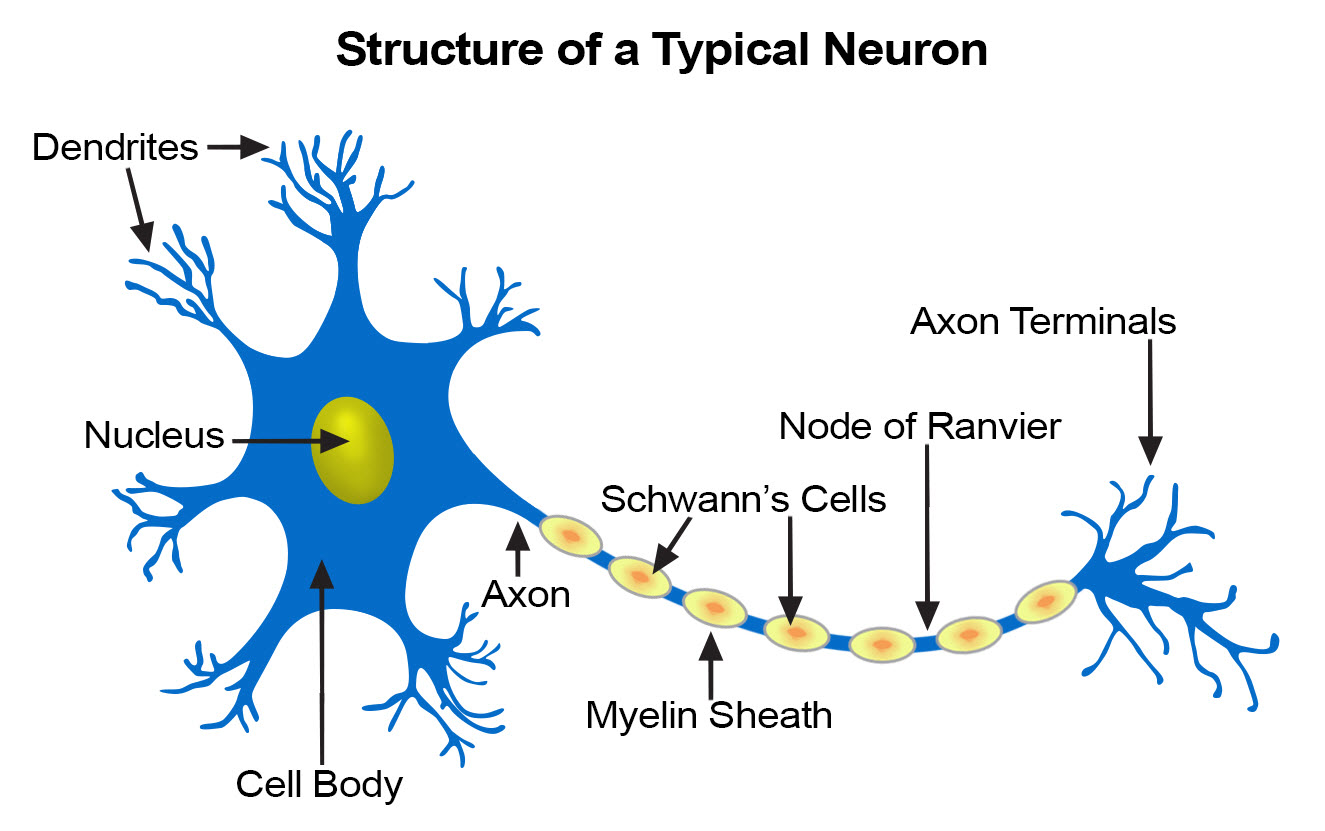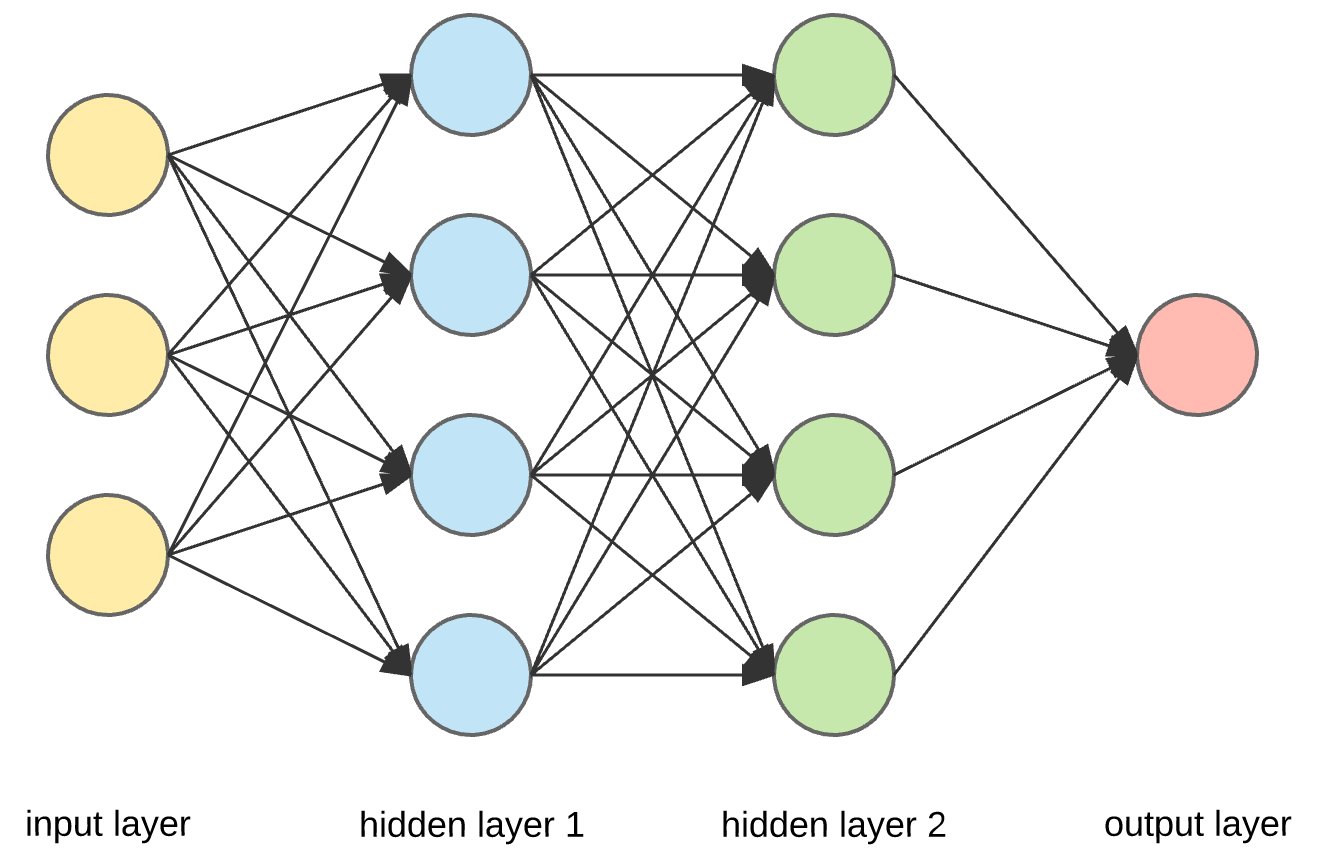# Understanding Artificial Neural network (ANN)

In the following tutorial, we will be understanding about artificial neural network.which is the backbone of machine learning and deep learning. in this fast-moving world, humans are trying to maximize production efficiency by involving machine learning and many algorithms related to this field

## Structure of ANN

The human brain system is composed of billion nerve cells called neurons. They are connected to other many cells by Axons. Stimuli from the external environment or inputs from sensory organs are accepted by dendrites(also know as receivers). These inputs create electric signals, which quickly travel through the neural network. A neuron can then send the message to other neurons to handle the issue or does not send it forward. this is how the basic structure of artificial neural network works.

Below image represents the basic structure of neuralbasic neuron structure

Another important advance has been the arrival of machine learning neural networks, in which different layers of a multilayer network system extract different features until it can recognize what it is looking for.this is called training a model on our parameter. which will be covered in the following part.

You might be interested in knowing: How to build a Feed Forward Neural Network in Python – NumPy

## Working of ANN

For a general idea about how a machine learning neural network trains, imagine a factory line. After the raw materials (the data set) are input, they are then passed down the to next part, with each subsequent stop or layer extracting a different set of high-level features. If the network is intended to recognize an object, the first layer might analyze the brightness of its pixels.

there are three layers of artificial neural network machine learning that are

• Input layer
• Hidden Layer
• Output layer

these three layers together make the structure of deep learning artificial neural network.ANN – Layers

in the above image, we can see that our data is been passed for input node that is our first layer of the network and then its been processed by hidden layer which applies various parameter based on our prediction system need, in above we have used two hidden layers for more complex deep learning. and finally, we have used the output layer to conclude our prediction

#### Conclusion: Artificial Neural Network

Hence we have covered the following  topic

• The basic structure of ANN
• working of ANN
• components of ANN

Hope you enjoyed this tutorial, see you in the next tutorial until then enjoy learning. In addition for more details please refer here.